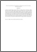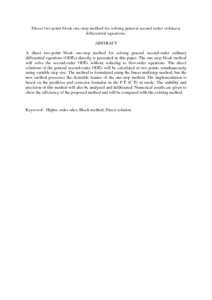# Direct two point block one-step method for solving general second order ordinary differential equations.

## Citation

Abdul Majid, Zanariah and Mokhtar, Nur Zahidah and Suleiman, Mohamed (2012) Direct two point block one-step method for solving general second order ordinary differential equations. Mathematical Problems in Engineering, 2012 (184253). pp. 1-16. ISSN 1024-123X

## Abstract

A direct two-point block one-step method for solving general second-order ordinary differential equations (ODEs) directly is presented in this paper. The one-step block method will solve the second-order ODEs without reducing to first-order equations. The direct solutions of the general second-order ODEs will be calculated at two points simultaneously using variable step size. The method is formulated using the linear multistep method, but the new method possesses the desirable feature of the one-step method. The implementation is based on the predictor and corrector formulas in the P E (C E) m mode. The stability and precision of this method will also be analyzed and deliberated. Numerical results are given to show the efficiency of the proposed method and will be compared with the existing method.Preview
PDF (Abstract)
Direct two point block one.pdfView Item